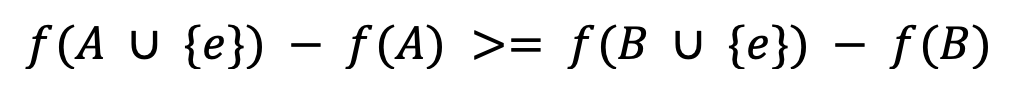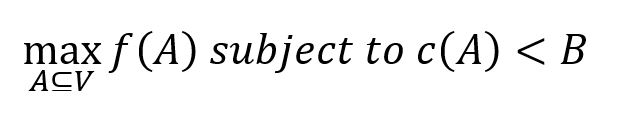# Change Nickname

Current Nickname:

Certifications

Certificate Issued at Valid until Serial No. File
Serial No. Valid until

Not having one? Apply now! >>>

Invoice

ProductName CreateTime ID Price File
ProductName CreateTime ID Price File

# CELF

## Overview

CELF (Cost Effective Lazy Forward) algorithm selects some seed nodes in a network as the propagation source to reach the effect of Influence Maximimzation (IM). CELF algorithm was proposed by Jure Leskovec et al. in 2007, it improves the traditional greedy algorithm based on the IC model by taking advantage of the submodularity. It only calculates the influence score of all nodes only at the initial stage and does not recalculate for all nodes afterwards, hence cost-effective. A typical application scenario of the algorithm is to prevent epidemic outbreaks by selecting a small group of people to monitor, so that any disease can be detected early in the outbreak.

Related materials of the algorithm:

## Basic Concepts

### IC Model

One common approach of studying a system is to abstract it into a mathematical model and study the model in replacement of studying the system itself, namely, simulation or modeling. For IM, one of the most frequently used models is Independent Cascade Model (IC Model).

IC Model is a probabilistic model, its basic architecture is as follows:

• When innitializing, non of nodes in the graph are activated, we choose a seed set and activate all nodes within the set.
• In the 1st round of spreading, seed nodes try to activate all their neighbors on the outbound edges with a certain probability for only one time, successfully or not.
• In following rounds of spreading, only nodes that were activated in previous round has the capability to activate, similarly, they try to activate neighbors on their outbound edges for only time for each round, successfully or not.
• When the number of nodes capable of activating in the graph is 0, the spreading ends.

When the spreading process finished, we are able to know the seed nodes' influence according to the amount of activated nodes in the graph. Ultipa's CELF algorithm adopts IC model to simulate the spreading process: given a probability `p` of activating (or spreading), for each time the nodes that are capable of activating try to active other nodes, the system selects a random number `random` within 0~1, the activation succeeds if `random < p`.

### Submodular

Submodularity is an attribute of aggregation function. It describes a phenomenon of diminishing Marginal Gain. For aggregation function `f()`, there's set `A ⊆ B ⊆ V` and element `e ∈ V-B`, it suggests to be submodular if conditions below are satisfied:It means that under the effect of `f()`, with a decreasing number of elements in the set, element `e`'s marginal gain is diminishing.

Function `IC()` that evaluate the seeds' influence is submodular, CELF improves the traditional greedy algorithm by taking advantage of the sobmodularity of `IC()`, saving tons of repetitive calculations with an efficiency improvement by 700 times compared to greedy algorithm, but they can give comparable results.

### Submodular Function Maximization

Influence Maximization problem can be regarded as a matter of sumodular function maximization which has following forms:Function `f()` is submodular, `A` is the input set, `c(A)` is a limit to `A` and it needs to be smaller than condition `B`. The limiting condition for this algorithm is a simple cardinal number, i.e, `c(A) = |A| < k`, and it requires to select subsets that include no more than a number `k` of elements to maximize the function result.

Sumodular function maximization is an NP-hard problem, and it only gives a near-optimal result.

## Special Cases

### Lonely node, Disconnected Graph

Lonely nodes are not connected to any other node, with 0 influence.

For unconnected graphs, the influence of nodes spread within each connected part.

### Self-loop Edge

A node is unable to activate other nodes via self-loop edges, so these edges do not affect the influence of nodes.

### Directed Edge

A node's influence is relevant to the number of their outbound edges.

## Command and Configuration

• Command: `algo(celf)`
• Configurations for the parameter `params()`:
Name
Type
Default
Specification
Description
seedSetSize int 1 >=1 The number of seed nodes
monteCarloSimulations int 1000 >=1 The number of Monte-Carlo simulations
propagationProbability float 0.1 (0,1) The probability of a node being activated

Example: Run CELF in the graph to find 3 seeds, the possibility of nodes being activated by their activated neighbors is 0.5, execute 1000 Monte-Carlo simulations

``````algo(celf).params({
seedSetSize: 3,
monteCarloSimulations: 1000,
propagationProbability: 0.5 }) as seeds
return seeds
``````

## Algorithm Execution

#### 1. File Writeback

Configuration
Data in Each Row
filename `_id`,`spread`

Example: Run CELF in the graph to find 3 seeds, the possibility of nodes being activated by their activated neighbors is 0.5, execute 1000 Monte-Carlo simulations, write the algorithm results back to file named seeds

``````algo(celf).params({
seedSetSize: 3,
monteCarloSimulations: 1000,
propagationProbability: 0.5
}).write({
file:{
filename: "seeds"}})
``````

#### 2. Property Writeback

Not supported by this algorithm.

#### 3. Statistics Writeback

This algorithm has no statistics.

### Direct Return

Alias Ordinal Type
Description
Column Name
0 []perNode Node and its marginal return `_uuid`, `spread`

Example: Run CELF in the graph to find 3 seeds, the possibility of nodes being activated by their activated neighbors is 0.5, execute 1000 Monte-Carlo simulations, define algorithm results as alias named seeds and return the results

``````algo(celf).params({
seedSetSize: 3,
monteCarloSimulations: 1000,
propagationProbability: 0.5 }) as seeds
return seeds
``````

### Streaming Return

Alias Ordinal Type
Description
Column Name
0 []perNode Node and its marginal return `_uuid`, `spread`

Example: Run CELF in the graph to find 3 seeds, the possibility of nodes being activated by their activated neighbors is 0.5, execute 1000 Monte-Carlo simulations, return seeds with their ID and createdTime properties as well as the score

``````algo(celf).params({
seedSetSize: 3,
monteCarloSimulations: 1000,
propagationProbability: 0.5 }).stream() as seeds
find().nodes({_uuid == seeds._uuid}) as nodes